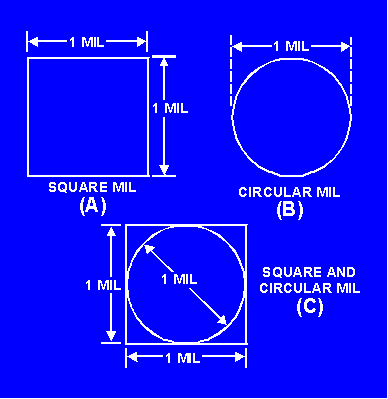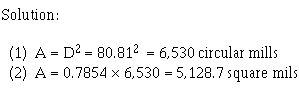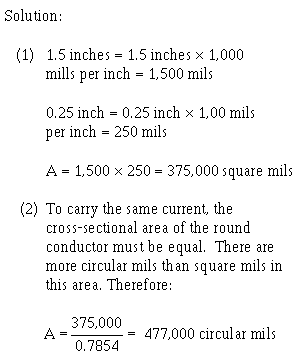Circular Mil electricity may be only a fraction of an inch. Therefore, it is convenient to express this diameter in mils to avoid using decimals. ">Custom SearchCIRCULAR MIL The circular mil is the standard unit of measurement of a round wire cross-sectional area (view C of figure 1-1). This unit of measurement is found in American and English wire tables. The diameter of a round conductor (wire) used to conduct electricity may be only a fraction of an inch. Therefore, it is convenient to express this diameter in mils to avoid using decimals. For example, the diameter of a wire is expressed as 25 mils instead of 0.025 inch. A circular mil is the area of a circle having a diameter of 1 mil, as shown in view B of figure 1-2. The area in circular mils of a round conductor is obtained by squaring the diameter, measured in mils. Thus, a wire having a diameter of 25 mils has an area of 252, or 625 circular mils. To determine the number of square mils in the same conductor, apply the conventional formula for determining the area of a circle (A = πr2). In this formula, A (area) is the unknown and is equal to the cross-sectional area in square mils, π is the constant 3.14, and r is the radius of the circle, or half the diameter (D). Through substitution, A = 3.14, and (12.5)2; therefore, 3.14 X 156.25 = 490.625 square mils. The cross-sectional area of the wire has 625 circular mils but only 490.625 square mils. Therefore, a circular mil represents a smaller unit of area than the square mil. Figure 1-2. - A comparison of circular and square mils.If a wire has a cross-sectional diameter of 1 mil, by definition, the circular mil area (CMA) is A = D2, or A = 12, or A = 1 circular mil. To determine the square mil area of the same wire, apply the formula A = πr2; therefore, A = 3.14 X (.5)2 (.5 representing half the diameter). When A = 3.14 X .25, A = .7854 square mil. From this, it can be concluded that 1 circular mil is equal to. 7854 square mil. This becomes important when square (view A of figure 1-2) and round (view B) conductors are compared as in view C of figure 1-2. When the square mil area is given, divide the area by 0.7854 to determine the circular mil area, or CMA. When the CMA is given, multiply the area by 0.7854 to determine the square mil area. For example, Problem: A 12-gauge wire has a diameter of 80.81 mils. What is (1) its area in circular mils and (2) its area in square mils?Problem: A rectangular conductor is 1.5 inches wide and 0.25 inch thick. What is (1) its area in square mils and (2) in circular mils? What size of round conductor is necessary to carry the same current as the rectangular bar?A wire in its usual form is a single slender rod or filament of drawn metal. In large sizes, wire becomes difficult to handle. To increase its flexibility, it is stranded. Strands are usually single wires twisted together in sufficient numbers to make up the necessary cross-sectional area of the cable. The total area of stranded wire in circular mils is determined by multiplying the area in circular mils of one strand by the number of strands in the cable. Q.5 Define a circular mil.Q.6 What is the circular mil area of a 19-strand conductor if each strand is 0.004 inch?Integrated Publishing, Inc. - A (SDVOSB) Service Disabled Veteran Owned Small Business